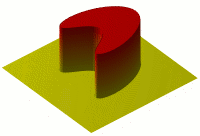# Partial differential equation facts for kids

Kids Encyclopedia FactsA visualisation of a solution to the two-dimensional heat equation with temperature represented by the third dimension

Partial Differential equations are a kind of mathematical equation. They are related to partial derivatives, in that obtaining an antiderivative of a partial derivative involves integration of partial differential equations.

## Related pagesPartial differential equation Facts for Kids. Kiddle Encyclopedia.